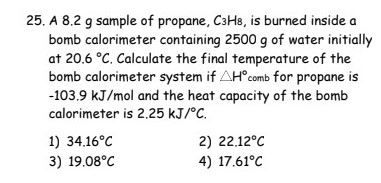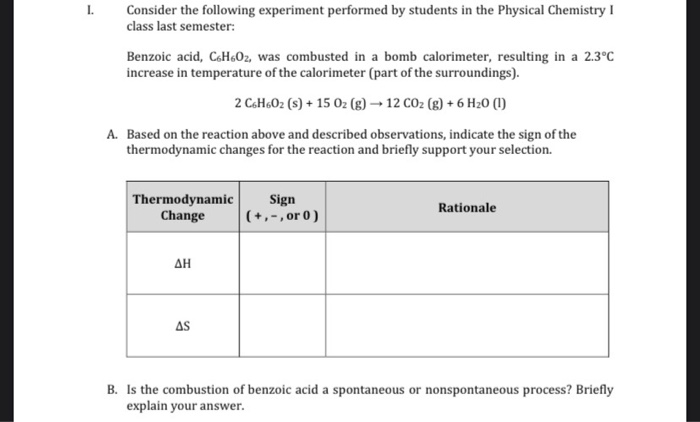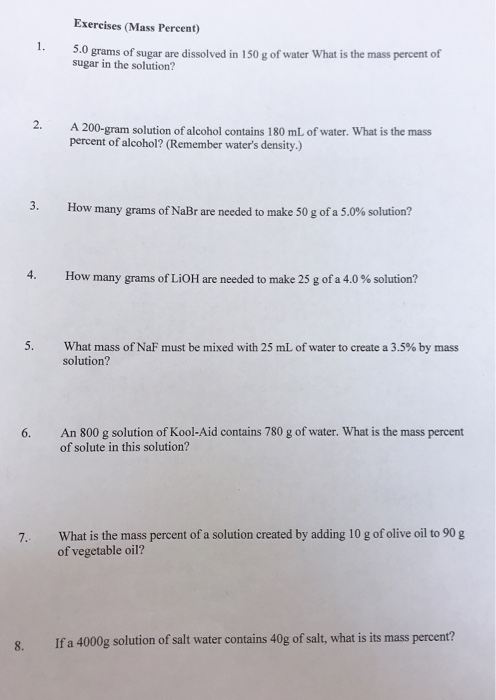Question

1. If 22.0 grams of propane (C3H8) is combusted in a bomb calorimeter containing 3.48 liters of water, calculate the enthalpy (ΔH) of propane if the water temperature rises 26.5 °C?f water to create a 3.5% by mass solution?

2. What mass of NaF must be mixed with 25 mL of water to create a 3.5% by mass solution?

heat gained by water(q) = m*s*DT

m = mass of water = d*v = 3480*1 = 3480 g

s = specific heat of water = 4.184 j/g.c

DT = 26.5

q = 3480*4.184*26.5 = 385.85 kj

No of mol of propane burned = 22.0/44 = 0.5 mol

DHcombustion of propane = -q/n = -385.85/0.5 = -771.7 kj/mol

2)
%(m/m) means , mass of solute present in 100 g solution.

mass percent of solution(m/m) = mass of solute/mass of solution*100

mass of solute(NaF) = x g

mass of solution = NaF + water

= x + 25      ( density of water = 1 g/ml)

3.5 = (x/(25+x))*100

x = 0.91

mass of solute(NaF) = x = 0.91 g

#### Earn Coins

Coins can be redeemed for fabulous gifts.

Similar Homework Help Questions
• ### 25. A 8,2 g sample of propane, C3H8, is burned inside a bomb calorimeter containing 2500...25. A 8,2 g sample of propane, C3H8, is burned inside a bomb calorimeter containing 2500 g of water initially at 20.6 °C. Calculate the final temperature of the bomb calorimeter system if AH comb for propane is -103.9 kJ/mol and the heat capacity of the bomb calorimeter is 2.25 kJ/°C. 1) 34.16°C 2) 22.12°C 3) 19.08°C 4) 17.61°C

• ### 1.14gm of Octane is combusted in bomb calorimeter, surrounded by 1000g of water. Initial temperature of...

1.14gm of Octane is combusted in bomb calorimeter, surrounded by 1000g of water. Initial temperature of water is 25C and final temperature is 33C. Heat Capacity of Calorimeter hardware (except for water) is 456 J/C . 2C8H18+25O2 --->16CO2+18H20 a)Calculate heat of solution and heat of calorimeter. b) Calculate net of reaction in KJ c)Determine molar enthalpy of combustion of octane in KJ/mol.

• ### if 22.0 mL of water containing 0.030 mol of HCl is mixed with 38.0 mL of...

if 22.0 mL of water containing 0.030 mol of HCl is mixed with 38.0 mL of water containing 0.030 mol of NaOH in a calorimeter such that the initial tempature of each solution was 27.0 degrees C and the final temperature of the mixture is 35.0 degrees C, how much heat (in kJ) is realeased in the reaction? Assume that the densities of the solutions are 1.00 g/mL.

• ### benzoic acid was combusted in a bomb calorimeter resulting in a 2.3 degree C incresse Consider...benzoic acid was combusted in a bomb calorimeter resulting in a 2.3 degree C incresse Consider the following experiment performed by students in the Physical Chemistry class last semester: Benzoic acid, C6H602, was combusted in a bomb calorimeter, resulting in a 2.3°C increase in temperature of the calorimeter (part of the surroundings). 2 C.H.O2 (s) + 15 O2(g) 12 CO2(g) + 6H20 (1) A. Based on the reaction above and described observations, indicate the sign of the thermodynamic changes for...

• ### A calorimeter contains 35.0 mL of water at 12.0 ∘C . When 2.30 g of X...

A calorimeter contains 35.0 mL of water at 12.0 ∘C . When 2.30 g of X (a substance with a molar mass of 70.0 g/mol ) is added, it dissolves via the reaction X(s)+H2O(l)→X(aq) and the temperature of the solution increases to 26.5 ∘C . Calculate the enthalpy change, ΔH, for this reaction per mole of X. Assume that the specific heat of the resulting solution is equal to that of water [4.18 J/(g⋅∘C)], that density of water is 1.00...

• ### A calorimeter contains 27.0 mL of water at 14.0 ∘C . When 2.00 g of X...

A calorimeter contains 27.0 mL of water at 14.0 ∘C . When 2.00 g of X (a substance with a molar mass of 77.0 g/mol ) is added, it dissolves via the reaction X(s)+H2O(l)→X(aq) and the temperature of the solution increases to 26.5 ∘C . Calculate the enthalpy change, ΔH, for this reaction per mole of X. Assume that the specific heat of the resulting solution is equal to that of water [4.18 J/(g⋅∘C)], that density of water is 1.00...

• ### A calorimeter contains 27.0 mL of water at 14.0 ∘C . When 2.00 g of X...

A calorimeter contains 27.0 mL of water at 14.0 ∘C . When 2.00 g of X (a substance with a molar mass of 77.0 g/mol ) is added, it dissolves via the reaction X(s)+H2O(l)→X(aq) and the temperature of the solution increases to 26.5 ∘C . Calculate the enthalpy change, ΔH, for this reaction per mole of X. Assume that the specific heat of the resulting solution is equal to that of water [4.18 J/(g⋅∘C)], that density of water is 1.00...

• ### A calorimeter contains 24.0 mL of water at 13.0 ∘C . When 2.00 g of X...

A calorimeter contains 24.0 mL of water at 13.0 ∘C . When 2.00 g of X (a substance with a molar mass of 64.0 g/mol ) is added, it dissolves via the reaction X(s)+H2O(l)→X(aq) and the temperature of the solution increases to 26.5 ∘C . Calculate the enthalpy change, ΔH, for this reaction per mole of X. Assume that the specific heat of the resulting solution is equal to that of water [4.18 J/(g⋅∘C)], that density of water is 1.00...

• ### Part A: A calorimeter contains 26.0 mL of water at 13.0 ∘C . When 2.10 g...

Part A: A calorimeter contains 26.0 mL of water at 13.0 ∘C . When 2.10 g of X (a substance with a molar mass of 49.0 g/mol ) is added, it dissolves via the reaction X(s)+H2O(l)→X(aq) and the temperature of the solution increases to 25.0 ∘C . Calculate the enthalpy change, ΔH, for this reaction per mole of X. Assume that the specific heat of the resulting solution is equal to that of water [4.18 J/(g⋅∘C)], that density of water...

• ### Exercises (Mass Percent) 1. 3.0 grams of sugar are dissolved in 150 g of water What...Exercises (Mass Percent) 1. 3.0 grams of sugar are dissolved in 150 g of water What is the mass percent of sugar in the solution? 2. A 200-gram solution of alcohol contains 180 mL of water. What is the mass percent of alcohol? (Remember water's density.) 3. How many grams of NaBr are needed to make 50 g of a 5.0% solution? 4. How many grams of LiOH are needed to make 25 g of a 4.0% solution? 5. What...